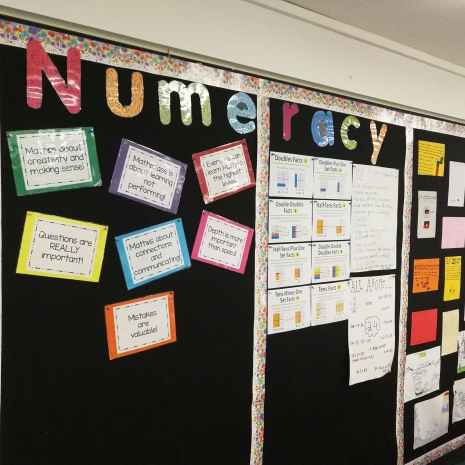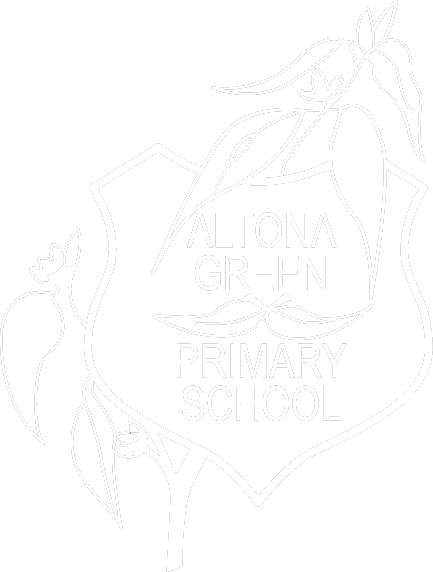# NumeracyLearning mathematics creates opportunities for and enriches the lives of all students. To ensure all of our students develop the knowledge, skills and dispositions to effectively engage with mathematics, our mathematics teaching and learning program is underpinned by the following: - We encourage problems to be solved with many different insights and strategies - We value mistakes as part of the process of learning - We create an environment where students approach mathematics with curiosity - We ensure students are given time to investigate ideas and grapple with problems - We ensure students connect, extend and explore mathematical ideas and concepts - We create many opportunities for mathematical dialogue - We link learning to real-life and authentic situations. - Maths is about making sense - We believe everyone can achieve at high levels The Mathematics curriculum is structured into the content strands (content, knowledge and skills) and proficiencies (thinking and doing of mathematics) of mathematics.
##### The Mathematics curriculum is structured into the content strands (content, knowledge and skills) and proficiencies (thinking and doing of mathematics) of mathematics.

Content Strands:

 Strands Number and Algebra Measurement and Geometry Statistics and Probability Sub-strands Number and place value Using units of measurement Chance Fractions and decimals Shape Data representation and interpretation Real numbers Geometric reasoning Money and financial mathematics Location and transformation Patterns and algebra Pythagoras and trigonometry Linear and non­-linear relationships

Proficiencies:

 Understanding Reasoning Fluency Problem Solving connect related ideas represent concepts in different ways identify commonalities and differences between aspects of content describe their thinking mathematically interpret mathematical information. explain their thinking deduce and justify strategies used and conclusions reached adapt the known to the unknown transfer learning from one context to another prove that something is true or false make inferences about data or the likelihood of events compare and contrast related ideas and explain their choices. make reasonable estimates calculate answers efficiently recognise robust ways of answering questions choose appropriate methods and approximations recall definitions and regularly use facts, can manipulate expressions and equations to find solutions. use mathematics to represent unfamiliar or meaningful situations design investigations and plan their approaches apply their existing strategies to seek solutions verify that their answers are reasonable.Our teachers carefully create mathematics lessons and units of work to ensure that all students develop the conceptual understanding and procedural fluency required for each of the content strands. We use robust assessment tools to determine each student’s current level of understanding and then collaboratively design learning programs to suit the needs of all individuals in each class. Our Mathematics curriculum also aims to ensure that students develop the understanding that Mathematics is composed of multiple but interrelated and interdependent concepts and systems. These concepts are applied beyond the mathematics classroom, such as Science, History and Technology.

### Contact Info

240 Victoria St Altona Meadows, Vic 3028

03 9360 0777
altona.green.ps@education.vic.gov.au

Monday - Friday: 8:30 am - 4:30 pm